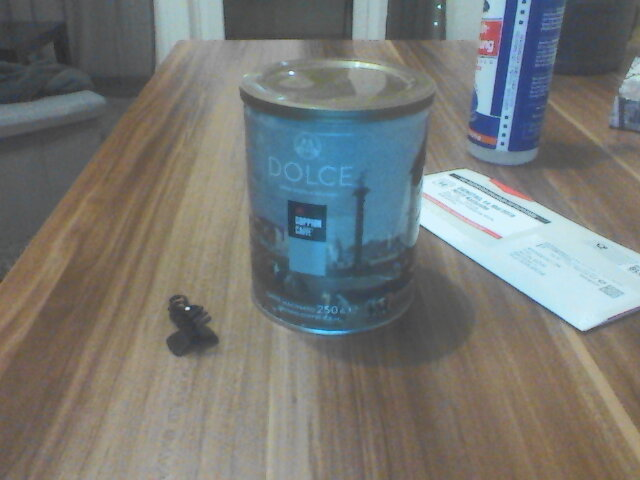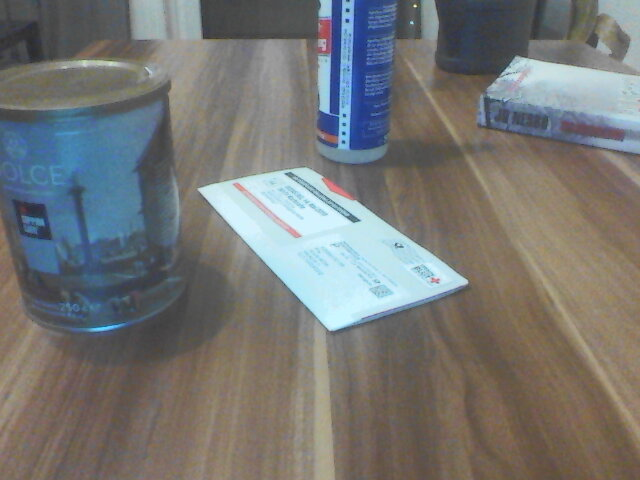# Harris Corner Detection

by Daniel Pollithy

This is an interactive demo for the Harris Corner Detector running in your browser with tensorflow.js.

The goal is to find points in an image which are corners. These points have Hessians with large, similar eigenvalues. If only one of the eigenvalues would be large, then the point might be part of an edge but not a corner.

Instead of calculating the eigenvalues (which is by the way not straight forward to implement in tensorflow.js) the authors suggested to calculate a score which is easier to obtain from the Hessian. They named it the Harris-Cornerness-function f.

$f = det(H) - kappa * trace^2 (H)$

det(H) is equal to the product of the eigenvalues. And trace(H) is equal to the sum of the eigenvalues.

Combined in the f value we get an indication about how different and how large the eigenvalues are.

See for more details: Wikipedia

In the case you are wondering what this is good for: I am attending multiple computer vision courses this semester (video analysis, automotive vision, deep learning for computer vision) which have some overlaps. In order to practice matrix operations and get some visuals I decided to build a collection of demos with tensorflow.js that implement the classic cv algorithms.

Note: Instead of the Hessian H the structure matrix M is used most of the times. (dy and dy are the gradient in x and y direction):

$\begin{pmatrix} dx^2 & dx \cdot dy \\ dy \cdot dx & dy^2 \end{pmatrix}$Harris Corner Settings:



/**
* Expands a gray value plane to a tensor which can be used by conv2d
*/
function expand_img(tf_img) {
return tf.expandDims(tf.expandDims(tf_img, axis=0), axis=-1)
}

/**
* Scales the values into [0, 1.0]
*/
function normalize_tensor(tf_img) {
var tf_new_image = tf_img.sub(tf.min(tf_img))
var img_span = tf_new_image.max().sub(tf_new_image.min())
tf_new_image = tf_new_image.div(img_span)
return tf_new_image
}

/**
* Approximate the gradient in x direction with the sobel filter x
*/
let sobel_x = [
[-1,0,1],
[-2,0,2],
[-1,0,1]
]
let tf_sobel_x = tf.tensor2d(sobel_x)
let expanded_filter_x = tf.expandDims(tf.expandDims(tf_sobel_x, axis=-1), axis=-1)
let img_deriv_x = tf.conv2d(tf_img, expanded_filter_x, [1,1], 'same', "NHWC" )
return img_deriv_x;
}

/**
* Approximate the gradient in y direction with the sobel filter y
*/
let sobel_y = [
[-1,-2,-1],
[0,0,0],
[+1,+2,+1]
]
let tf_sobel_y = tf.tensor2d(sobel_y)
let expanded_filter_y = tf.expandDims(tf.expandDims(tf_sobel_y, axis=-1), axis=-1)
let img_deriv_y = tf.conv2d(tf_img, expanded_filter_y, [1,1], 'same', "NHWC" )
return img_deriv_y;
}

/**
* Calculate Harris Corner detector
* https://en.wikipedia.org/wiki/Corner_detection#The_Harris_&_Stephens_/_Plessey_/_Shi%E2%80%93Tomasi_corner_detection_algorithms
*/
function harris(img, canvas_id, kappa, threshold){
// expand the image for tf.conv2d
let tf_img = expand_img(img)

// 2nd order gradients = hessian matrix

// hyperparameters
let kappa_ = tf.scalar(kappa);  // 0.04 - 0.15
let threshold_ = tf.scalar(threshold);  // 0.5

// determinant is the product of the eigenvalues

// trace is the sum of the eigenvalues

// this function is a surrogate to compare the magnitudes of the eigenvalues
let harris_cornerness_function = normalize_tensor(tf.sub(determinant, tf.mul(trace, trace).mul(kappa_)))

// threshold the points of interest
let harris_filtered = tf.greater(harris_cornerness_function, threshold_).asType('float32')

// add original image to background and clip the povs to 1.0
norm_img = tf.clipByValue(norm_img, 0.0, 1.0)

// display the result
tf.browser.toPixels(norm_img, document.getElementById(canvas_id))
}

/**
* Initialize code ASAP
*/
let left_img_element = document.getElementById('image_left');
let right_img_element = document.getElementById('image_right');

let img_left = tf.browser.fromPixels(left_img_element)
let img_right = tf.browser.fromPixels(right_img_element)

img_left = img_left.mean(2).div(255.0)
img_right = img_right.mean(2).div(255.0)

let height = left_img_element.height;
let width = left_img_element.width;

harris(img_left, "canvas_left", 0.005, 0.65);
harris(img_right, "canvas_right", 0.005, 0.65);

let kappa_slider = document.getElementById('kappa')
let threshold_slider = document.getElementById('threshold')

function updateImages() {
let kappa_value = parseFloat(kappa_slider.value)
let threshold_value = parseFloat(threshold_slider.value);

harris(img_left, "canvas_left", kappa_value, threshold_value);
harris(img_right, "canvas_right", kappa_value, threshold_value);
}

kappa_slider.onchange = updateImages
threshold_slider.onchange = updateImages
}